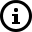# What is Sensitivity of an Accelerometer

An Accelerometer is a Sensor which has the ability to sense vibration acceleration. The SI unit of Acceleration is m/s^2. The Acceleration due to gravity is denoted as 'g'. The Acceleration due to gravity i.e 1 g is 9.81 m/s^2. Vibration Amplitude in acceleration is often represented in terms of g.Vibration Sensors such as Accelerometers are usually IEPE type and provide a voltage output proportional to acceleration.
This output is also called the sensitivity of the accelerometer. Since the Voltage is typically small, it is denoted as milli Volts or mV. Thus, the sensitivity of an accelerometer (its output) is denoted as mV/g.

Thus, when you buy a vibration sensor with a sensitivity of 100 mV/g, it means:
1. The Voltage output will be 100 mV peak when the acceleration is 1 g peak
2. The Voltage output will be 100 mV peak-peak when the acceleration is 1 g peak-peak
3. The Voltage output will be 100 mV RMS when the acceleration is 1 g RMS.
4. The Voltage output will be 200 mV peak when the acceleration is 2 g peak
The Sensitivity of an accelerometer does not remain fixed at all frequencies and at all temperatures. Thus, the sensitivity is reported at a nominal frequency of 80 Hz and at a temperature of 22 Deg Celsius. Usually, the sensitivity varies by +/- 5% over the frequency range and thus manufacturers report sensitivity as 100 mV/g +/- 5%

Based on the application, accelerometers of 50 mV/g or 500 mV/g output can also be used. However, 100 mV/g sensitivity is the most popular type of accelerometer used in vibration instruments and analysis.

Explore the Accelerometers offered by IRD Mechanalysis Limited